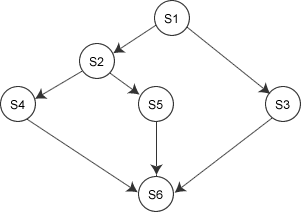``````wait(S){
while (S<=0);
S=S-1;
}
signal(S){
S=S+1;
}
``````

wait操作中，只要信号量S<=0，就会不断地测试。因此，该机制并未遵循“让权等待” 的准则，而是使进程处于“忙等”的状态。

``````typedef struct{
int value;
struct process *L;
} semaphore;
``````

``````void wait (semaphore S) { //相当于申请资源
S.value--;
if(S.value<0) {
block(S.L);
}
}
``````

wait操作，S.value--，表示进程请求一个该类资源，当S.value<0时，表示该类资源已分配完毕，因此进程应调用block原语，进行自我阻塞，放弃处理机，并插入到该类资源的等待队列S.L中，可见该机制遵循了“让权等待”的准则。

``````void signal (semaphore S) {  //相当于释放资源
S.value++;
if(S.value<=0){
remove a process P from S.L;
wakeup(P);
}
}
``````

signal操作，表示进程释放一个资源，使系统中可供分配的该类资源数增1，故S.value++。若加1后仍是S.value<=0，则表示在S.L中仍有等待该资源的进程被阻塞，故还应调用wakeup 原语，将S.L中的第一个等待进程唤醒。

``````semaphore S = 0;  //初始化信号量
P1 ( ) {
// …
x;  //语句x
V(S);  //告诉进程P2,语句乂已经完成
}
P2()）{
// …
P(S) ;  //检查语句x是否运行完成
y;  // 检查无误，运行y语句
// …
}
``````

``````semaphore S = 1;  //初化信号量
P1 ( ) {
// …
P(S);  // 准备开始访问临界资源，加锁
// 进程P1的临界区
V(S);  // 访问结束，解锁
// …
}
P2() {
// …
P(S); //准备开始访问临界资源，加锁
// 进程P2的临界区；
V(S);  // 访问结束，解锁
// …
}
````````````semaphore  al=a2=bl=b2=c=d=e=0;  //初始化信号量
S1() {
// …
V(al);  V(a2) ;  //S1已经运行完成
}
S2() {
P(a1);  //检查S1是否运行完成
// …
V(bl); V(b2); // S2已经运行完成
}
S3() {
P(a2);  //检查S1是否已经运行完成
// …
V(c);  //S3已经运行完成
}
S4() {
P(b1);  //检查S2是否已经运行完成
// …
V(d);  //S4已经运行完成
}
S5() {
P(b2);  //检查S2是否已经运行完成
// …
V(e);  // S5已经运行完成
}
S6() {
P(c);  //检查S3是否已经运行完成
P(d);  //检查S4是否已经运行完成
P(e);  //检查S5是否已经运行完成
// …;
}
``````

1) 关系分析。找出问题中的进程数，并且分析它们之间的同步和互斥关系。同步、互斥、前驱关系直接按照上面例子中的经典范式改写。

2) 整理思路。找出解决问题的关键点，并且根据做过的题目找出解决的思路。根据进程的操作流程确定P操作、V操作的大致顺序。

3) 设置信号量。根据上面两步，设置需要的信号量，确定初值，完善整理。

Last modification：April 1st, 2020 at 09:55 pm